Courses

Previous Year Questions: Motion in a Straight Line

28 Questions MCQ Test Physics For JEE | Previous Year Questions: Motion in a Straight Line

Description
Attempt Previous Year Questions: Motion in a Straight Line | 28 questions in 28 minutes | Mock test for JEE preparation | Free important questions MCQ to study Physics For JEE for JEE Exam | Download free PDF with solutions
QUESTION: 1

If a body losses half of its velocity on penetrating 3 cm in a wooden block, then how much will it penetrate more before coming to rest?  [AIEEE 2002]

Solution:

Let the initial velocity of the body at point A is v, AB is 3 cm.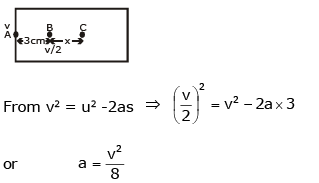On penetrating 3 cm in a wooden block, let the body moves x distance from B to C.
For B to C: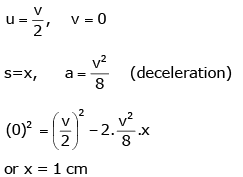Note: Here, it is assumed that retardation is uniform.

QUESTION: 2

From a building, two balls A and B are thrown such that A is thrown upwards and B downwards (both vertically with the same velocity). If vA and vB are their respective velocities on reaching the ground, then:    [AIEEE 2002]

Solution:

From the conservation of energy, potential energy at height h = kinetic energy at the ground.
Therefore, at height h, PE of ball A, PE = mAgh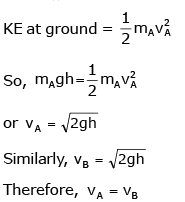QUESTION: 3

A ball whose kinetic energy is E is projected at an angle of 45° to the horizontal. The kinetic energy of the ball at the highest point of its flight will be:          [AIEEE 2002]

Solution:

At the highest point of its flight, the vertical component of velocity is zero and the only horizontal component is left which is ux = u cosθ
Given θ = 450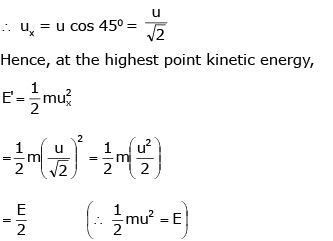QUESTION: 4

Speeds of two identical cars u and 4u at a specific instant. The ratio of the respective distances at which the two cars are stopped from that instant by the same braking force:

[AIEEE 2002]

Solution:

In this question, the cars are identical means the coefficient of friction between the tyre and the ground is the same for both the cars, as a result, retardation is the same for both the cars equal to mg.
Let first car travel distance s1, before stopping while second car travel distance s2, then from v2 = u2 - 2as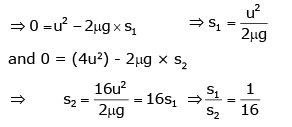QUESTION: 5

The coordinates of a moving particle at any time t are given by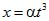and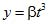. The speed of the particle at time t is given by:

[AIEEE 2003]

Solution: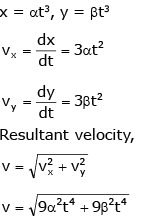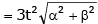QUESTION: 6

A boy playing on the roof of a 10 m high building throws a ball with a speed of 10 m/s at an angle of 30° with the horizontal. How far from the throwing point will the ball be at the height of 10 m from the ground? [g = 10 m/s2, sin 30° = 1/2, cos 30° = √3/2]

[AIEEE 2003]

Solution:

We have to simply calculate the range of the projectile.
► R = u2sin2θ/g
► R = (10)2 * sin (2 * 30º) / 10
► R = 10 * (√3/2) = 8.66 m

QUESTION: 7

A car moving with a speed of 50 km/h, can be stopped by brakes after at least 6 m. If the same car is moving at a speed of 100 km/h, the minimum stopping distance is:

[AIEEE 2003]

Solution:

We know that retardation of a car with an initial speed of 50kmph stops after 6 m.
We know that v2 - u2 = 2as  (third equation of motion)
► 0 - 502 = 2 * a * 6
► a = - 502 / 12
Hence when the initial speed is 100 kmph
We get,
► -1002 = 2 * a * s
► 2 * s = 12 * (1002 / 502)
Thus we get s = 24 m.

QUESTION: 8

A ball is thrown from a point with a speed v0 at an angle of projection θ. From the same point and at the same instant, a person starts running with a constant speed v0 / 2 to catch the ball. Will the person be able to catch the ball? If yes, what should be the angle of projection?

[AIEEE 2004]

Solution:

For a person to catch the ball they should travel the same distance along the horizontal axis in time T.
Distance covered by person, R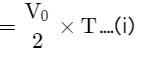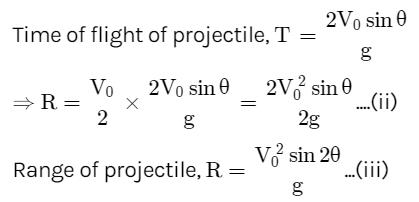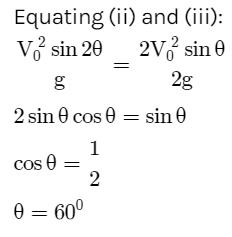QUESTION: 9

A particle is acted upon by a force of constant magnitude which is always perpendicular to the velocity of the particle. The motion of the particle takes place in a plane, it follows that:

[AIEEE 2004]

Solution:

When a force of constant magnitude acts on the velocity of a particle perpendicularly, then there is no change in the kinetic energy of the particle. Hence, kinetic energy remains constant.

QUESTION: 10

An automobile travelling at a speed of 60 km/h, can apply the brake to stop within a distance of 20 m. If the car is going twice as fast, i.e. 120 km/h, the stopping distance will be:

[AIEEE 2004]

Solution:
• We know that with retardation of a car with an initial speed of 60 kmph stops after 20m.
• We know that v2 = 2as  (third equation of motion). Thus we get that for some constant retardation, stopping distance is proportional to the square of the initial speed.
• Hence when the initial speed is 120kmph we get,
s = 20 x (120/60)2
s = 80 m
QUESTION: 11

A ball is released from the top of a tower of height h meter. It takes T seconds to reach the ground. What is the position of the ball in T/3 seconds?

[AIEEE 2004]

Solution:

Second law of motion gives: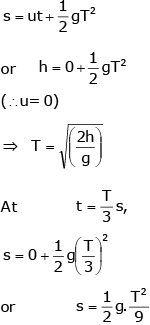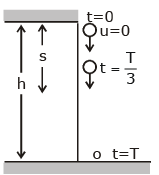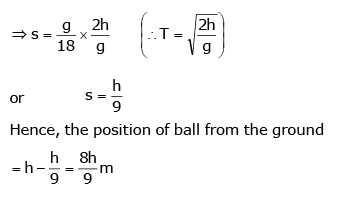QUESTION: 12

A parachutist after bailing outfalls 50 m without friction. When the parachute opens, it decelerates at 2 ms-2. He reaches the ground with a speed of 3 ms-1. At what height, did he bail out?

[AIEEE 2005]

Solution:

Parachute bails out at height H from ground. Velocity at A: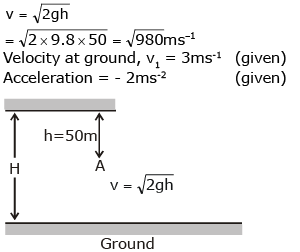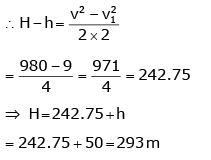QUESTION: 13

A particle is moving eastwards with a velocity of 5 ms-1. In 10 s the velocity changes to 5 ms-1 northwards. The average acceleration in this time is:

[AIEEE 2005]

Solution: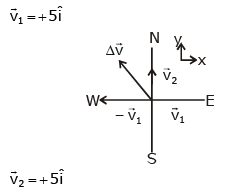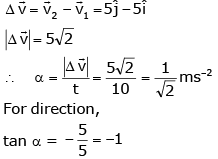The average acceleration is 1/√2 ms-2 towards the northwest.

QUESTION: 14

A car, starting from rest, accelerates at the rate f through a distance s, then continues at a constant speed for time t and then decelerates at the rate f/2 to come to rest. If the total distance travelled is 15 s, then:

[AIEEE 2005]

Solution:

The velocity-time graph for the given situation can be drawn as below. Magnitudes of the slope of OA = f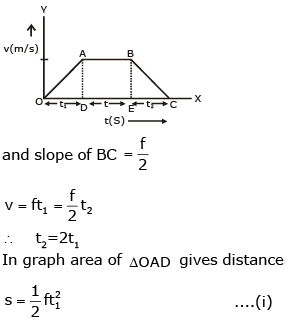Area of rectangle ABED gives distance travelled in time t.
► s2 = (ft1) * t
Distance travelled in time t2,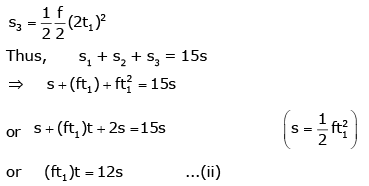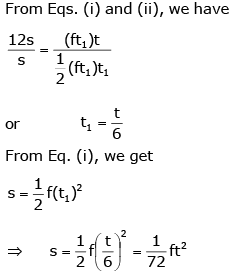QUESTION: 15

The relation between time t and distance x is t = ax2 + bx, where a and b are constants. The acceleration is:

[AIEEE 2005]

Solution:

Given, t = ax+ bx
Differentiating w.r.t  t, we get: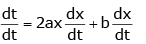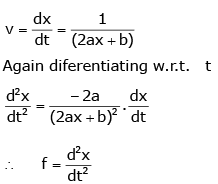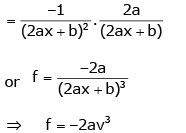QUESTION: 16

A projectile can have the same range R for two angles of projection. If t1 and tbe the times of flights in the two cases, then the product of the two times of flights is proportional to:

[AIEEE 2005]

Solution:

A projectile can have the same range if the angle of projection are complementary ie, θ and (90- θ). Thus, in both cases: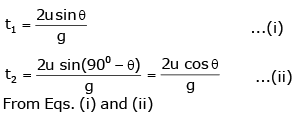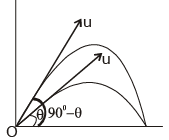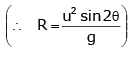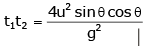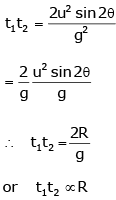QUESTION: 17

A particle located at x = 0 at time t = 0, starts moving along the positive x-direction with a velocity v that varies as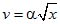. The displacement of the particle varies with time as:

[AIEEE 2006]

Solution: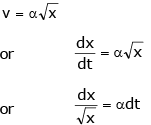On performing integration: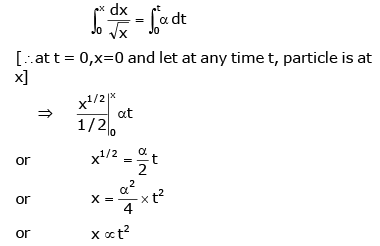QUESTION: 18

A particle is projected at 60° to the horizontal with a kinetic energy K. The kinetic energy at the highest point is:

[AIEEE 2007]

Solution:

Kinetic energy at highest point,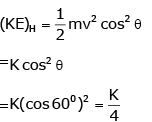QUESTION: 19

The velocity of a particle is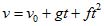. If its position is x = 0 at t = 0, then its displacement after unit time (t = 1) is:

[AIEEE 2007]

Solution:

v = v​+ gt + ft2
0x​ dx = ∫01​(v0​ + gt + ft2) dt
► x = v0 ​+ g/2 ​+ f​/3

QUESTION: 20

A body is at rest at x = 0. At t = 0, it starts moving in the positive x-direction with a constant acceleration. At the same instant, another body passes through x = 0 moving in the positive x-direction with a constant speed. The position of the first body is given by x1 (t) after time t and that of the second body by x2(t) after the same time interval. Which of the following graphs correctly describes (x1 – x2) as a function of time?

[AIEEE 2008]

Solution: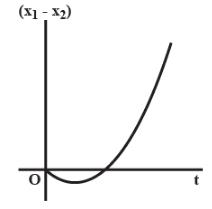► x1​(t) = 1​/2 at2
► x2​(t) = vt
► (x1 - x2) = 1/2​ at− vt
Now Plot (x1​−x2) vs t

QUESTION: 21

Consider a rubber ball freely falling from a height h = 4.9 m onto a horizontal elastic plate. Assume that the duration of the collision is negligible and the collision with the plate is totally elastic. Then the velocity as a function of time the height as a function of time will be:
[AIEEE 2009]

Solution:
• We know, v = u + gt
As the ball is dropped, v = gt.
When coming down, v increases then makes collision, the value of v becomes +ve, then value of v decreases, becomes zero and then increases.
• The change from +v to –v is almost instantaneous. Using -ve signs when coming down, so option c is correct.
Further h = (1/2) gt2  is a parabola. Therefore (c) is correct.
QUESTION: 22

A particle has initial velocity (2i + 3j) and acceleration (0.3i + 0.2j). The magnitude of velocity after 10 s will be:

Solution:

From the equation of motion, we knows,
v = u + at  ...(1)
► u = (2i + 3j)
► a = (0.3i + 0.2j)
► t = 10 s
On putting these values in first equation we get:
v = (2i + 3j) + (0.3i + 0.2j) * 10
v = 5i + 5j
|v| = 5√2

QUESTION: 23

A small particle of mass m is projected at an angle θ with the x-axis with an initial velocity v0 in the x-y plane as shown in the figure. At a time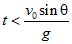, the angular momentum of the particle is:

[AIEEE 2010]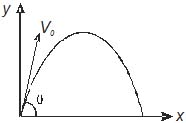Solution: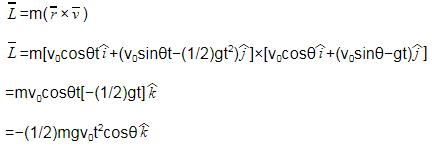QUESTION: 24

A particle is moving with velocity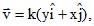where k is a constant. The general equation for its path is:

[AIEEE 2010]

Solution:

As, v = k(yi + xi)
vx = Ky → dx/dt = Ky
v= Kx → dy/dt = Kx
Dividing both equations,
► dy/dx = dy/dt divided by dx/dt = Kx / Ky = x/y
► ydy = xdx
Integrating the equation,
► y2/ 2 = x2/2 + c
► y= x+ constant

QUESTION: 25

A water fountain on the ground sprinkles water all around it. If the speed of water coming out of the fountain is v, the total area around the fountain that gets wet is:

[AIEEE 2011]

Solution:

The maximum range of water coming out of the fountain,
Rm = v2/g
Total area around fountain,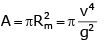QUESTION: 26

An object, moving with a speed of 6.25 m/s, is decelerated at a rate given by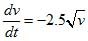where v is the instantaneous speed. The time taken by the object, to come to rest, would be:

[AIEEE 2011]

Solution: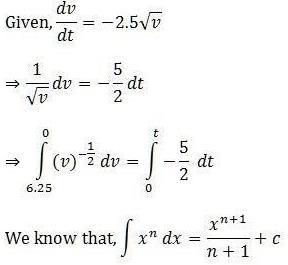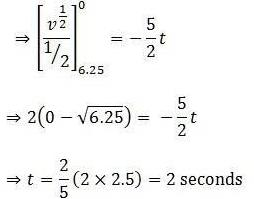QUESTION: 27

A boy can throw a stone up to a maximum height of 10 m. The maximum horizontal distance that the boy can throw the same stone up to will be:

[AIEEE 2012]

Solution:

Maximum speed with which the boy can throw stone is: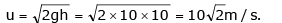Range is maximum when the projectile is thrown at an angle of 450 thus,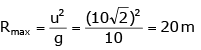QUESTION: 28

A particle of mass m is at rest at the origin at time t = 0. It is subjected to a force F(t) = F0e-bt in the x-direction. Its speed v(t) is depicted by which of the following curves?

[AIEEE 2012]

Solution:

As the force is exponentially decreasing, so its acceleration, i.e., rate of increase of velocity will decrease with time. Thus, the graph of velocity will be an increasing curve with decreasing slope with time.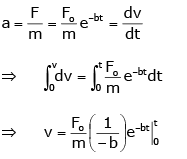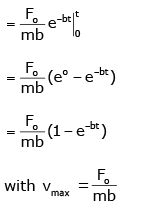Use Code STAYHOME200 and get INR 200 additional OFF Use Coupon Code

Track your progress, build streaks, highlight & save important lessons and more!

Similar ContentRelated tests